# Using the Law of Cosines to Find an Unknown Angle

The Law of Cosines states:

c2 = a2 + b2 - 2ab cosC

In which c is the side across from angle C.

Remember that the Law of Cosines works for any triangle, not just right triangles.

In the last lesson we used the Law of Cosines to find the length of the third side of a triangle when you know the length of two sides and the angle in between.

This law can also be used to find the measure of an angle when the three sides of a triangle are given.

To use the Law of Cosines to find an unknown angle:

1. Identify c as the side across from the angle you are trying to find.
2. Substitute the values into the Law of Cosines.
3. Solve the equation for angle C.

Examples:

1. Find the measure of angle X.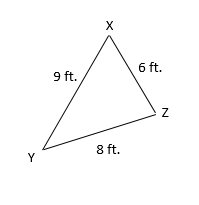First we must identify our sides. We are looking for angle X, thus c = 8 (because the side across from angle X is 8 ft.)

Let's substitute our values into the formula: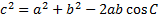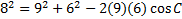Now we need to simplify the equation and solve for C: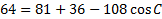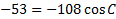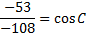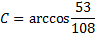Carefully input this into your calculator. Remember that the arccos button may look like this: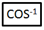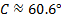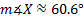2.  Find the measure of angle Y (using the same triangle in the example above).
Now that we know the measure of angle X, we could use the Law of Sines to find the measure of angle Y. However, if we made a mistake on angle X, then our answer for angle Y would also be incorrect. So let's use the Law of Cosines again:

First we must identify our sides. Since we are now looking for angle Y, c = 6 (because the side across from angle Y is 6 ft.)
Let's substitute our values into the formula: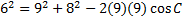Now we need to simplify the equation and solve for C: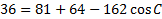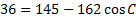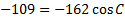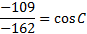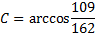Carefully input this into your calculator.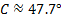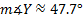Practice: Use the Law of Cosines to find the angle given. Round your answer to the nearest tenth. For #1-2, use the picture given.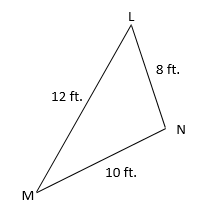1. Find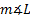.

2. Find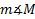.

3. Find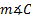if a = 4 ft., b = 9 ft., c = 6 ft.

4. Find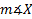if x = 5 in., y = 7 in., z = 9 in.

5. Find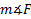if d = 10 cm. e = 8 cm., f = 7 cm.

Answers: 1) 55.8° 2) 41.4° 3)32.1° 4) 33.6° 5) 44.0°

 Related Links: Math Fractions Factors